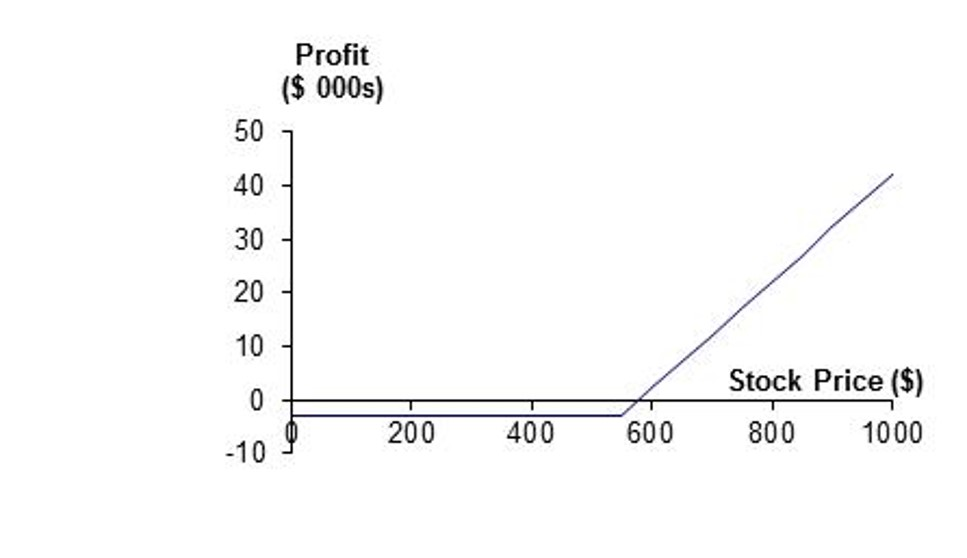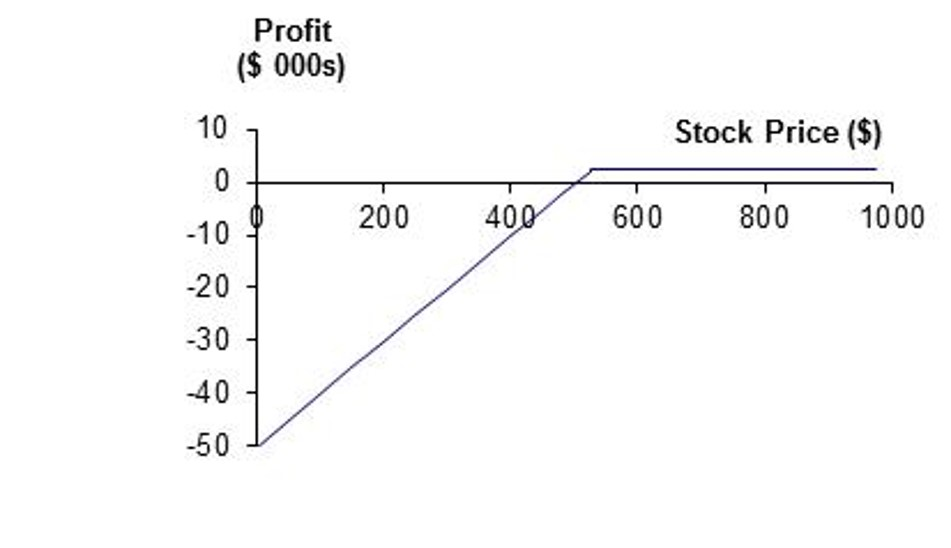# Chapter 3 W4 Class

## 3.1 OptionsDo you know what kind of option they are?

First: Long Call; Second: Short Put

Definition

1. A call is an option to buy
2. A put is an option to sell
3. European option: Exercisable only at expiration
4. American option: Exercisable at any time

1. Long Call
2. Long Put
3. Short Call
4. Short Put

### 3.1.2 Specification of Options

1. Expiration Date
2. Strike Price X
3. E or A
4. C or P

### 3.1.3 Moneyness:

• At-the-money
• In-the-money
• Out-of-the money option
 $$c$$: European call option price $$C$$ American call option price $$p$$: European put option price $$P$$ American put option price $$S_0$$: Stock Price today $$S_T$$ Stock price at option maturity $$K$$: Strike price $$D$$ PV of dividends during option’s life $$T$$: Life of option $$r$$ risk-free rate for maturity T with cts compounding $$\sigma$$: Volatility of stock

### 3.1.4 Stock Split

Suppose you own options with a strike price of K to buy (or sell) N shares: - No adjustments are made to the option terms for cash dividends - When there is an n-for-m stock split, - the strike price is reduced to mK/n - the no. of shares that can be bought (or sold) is increased to nN/m - Stock dividends are handled in a manner similar to stock splits

### 3.1.5 Market Makers

• Most exchanges use market makers to facilitate options trading
• A market maker quotes both bid and ask prices when requested
• The market maker does not know whether the individual requesting the quotes wants to buy or sell ### Margin
• Margin is required when options are sold (Example:A total of 100% of the proceeds of the sale plus 10% of the underlying share price (call) or exercise price (put))

### 3.1.6 Other information

• Warrants: options that are issued by a corporation or a financial institution
• Employee stock options: Employee stock options are a form of remuneration issued by a company to its executives

### 3.1.7 Effect of Variables on Option Pricing

Var c p C P
$S_0$ + - + -
$K$ - + - +
$T$ ?? ?? + +
$\sigma$ + + + +
$r$ + - + -
$D$ - + - +

Explanation:

• Keep other things unchanged, a higher S0 will be more likely to pass K, thus it’ll be price higher. Other logics follow

• T for European options are murky. Since it’s exercisable only at a certain date, you never know what happens closer or on that date. The story is totally different in American options.

### 3.1.8 Option Bounds

• An American option is worth at least as much as the corresponding European option C>=c; P>=p
Case Eur opean call Eur opean put Ame rican Put-call Parity
Basic c>=m ax(So - Xe ^(-r T),0) p> =max( Xe^( -rT)- So,0) S0 - X < C - P < S0 - Xe^ (-rT) c + Xe^(-rT) = p + S0
Discrete Dividend c ≥ m ax(S0 -D-Xe ^(-r T),0) p ≥ m ax(D+ Xe^( -rT)- S0,0) S0 - D - X < C - P < S0 - Xe^( –rT) c + D + Xe^(-rT) = p + S0
Dividend Yield c ≥ max( S0e^ (-qT) - Xe^( -rT), 0) p ≥ max (Xe^ (-rT) – S 0e^( -qT), 0) - c+ X e^(-rT)=p + S0e^(-qT)
Futures Options c ≥ m ax((F 0-X)e ^(-r T),0) p ≥ m ax((X -F0)e ^(-r T),0)

F0e
^(-rT )-X< C-P < F0- Xe^( -rT)

C ≥F0-X and P ≥X-F0

c+ X e^(-rT)=p + F0e^(-rT)